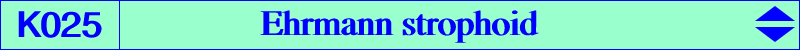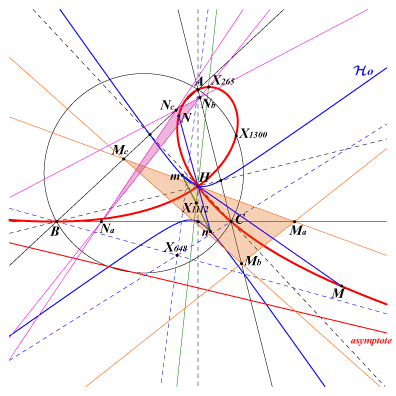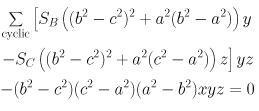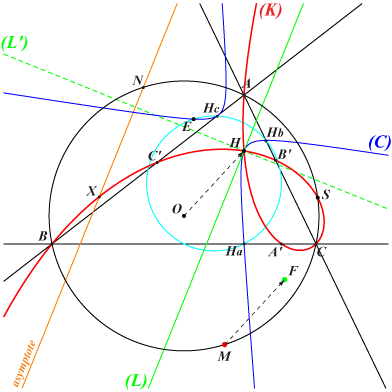X(4), X(30), X(265), X(316), X(671), X(1263), X(1300), X(5080), X(5134), X(5203), X(5523), X(5962), X(10152), X(11604), X(11605), X(11703), X(13495), X(16172), X(19552), X(31862), X(31863), X(34150), X(34169), X(34170), X(34171), X(34172), X(34173), X(34174), X(34175), X(34239), X(34240), X(37888), X(38945), X(38946), X(38947), X(38948), X(38949), X(38950), X(38951), X(38952), X(39158), X(39159), X(39160), X(39161), X(39985), X(39989), X(39990), X(39991), X(39992), X(39993), X(41521), X(42809), X(42810), X(47103), X(47104), X(47105), X(47106), X(47107), X(47108), X(47109), X(47110), X(47111) foci of the Steiner circum-ellipse Let A'B'C' be the circumcevian triangle of H. The points A1 = BC /\ A'X(30) and A2 = AX(1300) /\ A'A1lie on K025. B1, B2 and C1, C2 are defined likewise. ***Two points M and N have equal length cevians (i.e. AMa = ANa, etc) if and only if equivalently : they are two conjugated points on the Ehrmann strophoid. they are the antigonal conjugates of two points of the Euler line which are inverse with respect to the circumcircle (Jean-Pierre Ehrmann). they are the orthoassociates (inverses with respect to the polar circle) of two antipodes m and n on the rectangular hyperbola Ho which is the Feuerbach hyperbola of the orthic triangle when ABC is acute-angled and a Boutin hyperbola when ABC is obtuse-angled (Jean-Pierre Ehrmann). See a generalization at K186. The bisectors of (FM,FN) are parallel and perpendicular to the Euler line. Ho is the isogonal transform (with respect to the orthic triangle) of the Euler line of ABC. It contains the vertices of the cevian triangles of H = X(4) and X(648) = trilinear pole of the Euler line and the triangle centers X(4), X(6), X(52), X(113), X(155), X(185), X(193), X(1162), X(1163), X(1829), X(1839), X(1843), X(1858), X(1986). Its center is X(1112). Its equation is : (b^2 - c^2)(SA^2 x^2 + SB SC y z) + cyclic = 0. See also Clark Kimberling, Conics Associated with a Cevian Nest, Forum Geometricorum, vol. 1 (2001), 141 -- 150. K025 contains the foci of the Steiner circum-ellipse since the six cevians of these two points all have the same length. The tangents to the strophoid at H are parallel to the asymptotes of the Jerabek hyperbola : they are the Steiner lines of the points where the Euler line meets the circumcircle. The singular focus is F = X(265), isogonal conjugate of the inversive image of H in the circumcircle. The real asymptote is parallel to the Euler line and passes through X(110), X(477) and the reflection X(7728) of F in H. It meets K025 again at X(39985) also on the line X(4)X(526). Note that X(39985) is the antigonal image of X(15329), a point on the Euler line which is the center of the circle (C), orthogonal to the circumcircle and passing through X(110), X(476). If M, N are two points on the Euler line and inverse in (C) then their antigonal images M', N' are two points on K025 on a line parallel to the Euler line. *** A construction : A variable line L passing through the singular focus F = X(265) meets the Euler line at P. The circle with center P passing through the orthocenter H of ABC meets the line L at two points on the cubic. *** Locus properties : K025 is the antigonal image of the Euler line (See Hyacinthos #7847 for references and also K186, K288, K289). See below for a generalization. K025 is the isogonal transform of the Jerabek strophoid. K025 is the anticomplement of K038, the Stammler strophoid. K025 is the inversive image of the rectangular hyperbola which passes through H, X(54), X(110), X(2574), X(2575), the vertices Ah, Bh, Ch of the circumcevian triangle of H under the inversion which swaps the vertices of ABC and those of AhBhCh. K025 is the locus of P such that the reflections of AP, BP and CP through the altitudes of ABC are concurrent in a point P* also on the cubic. Thus, P and P* can be reflected via A, B, C in the Steiner circum-ellipse as an elliptic mirror. K025 is the locus of Q common point of the circles BCA', CAB', ABC' where A', B', C' are the reflections of A, B, C in a point of the Euler line (Jean-Pierre Ehrmann). K025 is the locus of pivots of circular pKs which pass through the orthocenter. See CL035. Let P be a point and PaPbPc its pedal triangle. A1 is the projection of A on PPa and A2 the reflection of P in A1. Define B2, C2 similarly. ABC and A2B2C2 are perspective if and only if P lies on K025. The perspector also lies on K025. Locus of P such that P, H and the DF-pole of P are collinear. See CL039. K025 is the locus of pivots of circular pKs whose orthic line passes through the nine point center. See CL035. K025 is the pedal curve, with respect to H, of the anticomplement of the Kiepert parabola.Generalization of property 5 This can be found in Hyacinthos messages #1811, 1812, 1816, 1821, 1822, 1823, 1831, 1833 where H is replaced by a point R and the altitudes by the cevian lines of R. In general, we obtain a circular nodal cubic with node R and the nodal tangents are always perpendicular. When R is an in/excenter, the locus is the whole plane. When R lies on the circumcircle, the cubic decomposes into the circumcircle and a line through O. When R lies at infinity, the cubic decomposes into the line at infinity and a circum-rectangular hyperbola. When R = O, we obtain the Jerabek strophoid K039. For other circum-strophoids passing through H (or any given point), see K165 and Q038. K025 and K039 are members of the class CL038 of non-isogonal circum-strophoids. See another generalization and other related cubics in Table 43. The reflection of K025 about X(5) is K725.Generalization of property 1 Let (L) be a line through the orthocenter H of ABC which can be seen as the Steiner line of a point N on the circumcircle (O). The antigonal transform (K) of (L) is a circular nodal circum-cubic with node H.M is the isogonal conjugate of the infinite point of (L) and the antipode of N on (O). (C) is the bicevian conic passing through H and the vertices of the orthic triangle which is tangent at H to (L). It is the orthoassociate of (K) i.e. its inverse in the polar circle. E is the complement of M, a point on the nine point circle and on (C). F is the singular focus of (K). It is the image of M under the translation that maps O onto H, hence F is a point on the circle C(H, R). S is the last point of (K) on (O). It is the orthoassociate of E. The nodal tangents at H to (K) are parallel to the asymptotes of (C). The real asymptote of (K) is the parallel at N to (L).The following table gives a selection of remarkable cubics (K). (L) is the line passing through H and P.P (K) or centers X(i) on (K) for i = centers X(i) on (C) for i = F S E remarks X(2) K025 4, 6, 52, 113, 155, 185, 193, etc X(265) X(1300) X(113) strophoid X(523) K186 4, 125 X(7728) X(107) X(125) nK X(6) K288 4, 25, 51, 132, 1842, 1859 X(10749) X(98) X(132) K0 X(69) K289 4, 114, 427, 1899 X(6321) X(3563) X(114) X(1) 4, 80, 515, 5176, 5179, 7424, 11608 4, 19, 46, 65, 117, 208, 407, etc X(10747) X(32706) X(117) X(8) 4, 517, 915, 1320, 1325, 5011, 11611 4, 119, 429, 1826, 1837, 1863, etc X(10738) X(915) X(119)